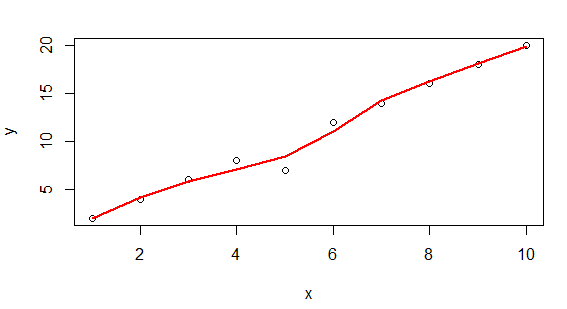2 views

I'm trying to draw a smooth curve in R. I have the following simple toy data:

> x

  1  2  3  4  5  6  7  8  9 10

> y

  2  4  6  8  7 12 14 16 18 20

Now when I plot it with a standard command it looks bumpy and edgy, of course:

plot(x,y, type='l', lwd=2, col='red')

How can I make the curve smooth so that the 3 edges are rounded using estimated values? I know there are many methods to fit a smooth curve but I'm not sure which one would be most appropriate for this type of curve and how you would write it in R.

by

To fit a smooth curve to the data, you can use the loess() function that fits a polynomial surface determined by one or more numerical predictors, using local fitting.

x <- c(1  ,2,  3,  4,  5,  6,  7,  8,  9, 10)

y <- c(2,  4,  6,  8,  7, 12, 14, 16, 18, 20)

ls <- loess(y~x)

plot(x,y)

lines(predict(ls), col='red', lwd=2)

Output: